# ACT Math : How to find the length of the side of an acute / obtuse triangle

## Example Questions

### Example Question #1 : How To Find The Length Of The Side Of An Acute / Obtuse Triangle

A triangle has a perimeter of 36 inches, and one side that is 12 inches long. The lengths of the other two sides have a ratio of 3:5. What is the length of the longest side of the triangle?

8

15

14

9

12

15

Explanation:

We know that the perimeter is 36 inches, and one side is 12. This means, the sum of the lengths of the other two sides are 24. The ratio between the two sides is 3:5, giving a total of 8 parts. We divide the remaining length, 24 inches, by 8 giving us 3. This means each part is 3. We multiply this by the ratio and get 9:15, meaning the longest side is 15 inches.

### Example Question #1 : How To Find The Length Of The Side Of An Acute / Obtuse Triangle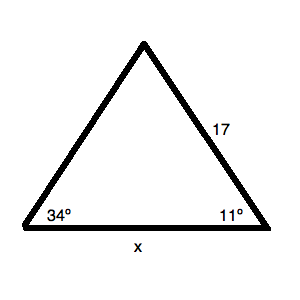What is the value ofin the triangle above? Round to the nearest hundredth.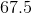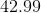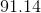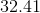Cannot be computedExplanation:

What is the value ofin the triangle above? Round to the nearest hundredth.

Begin by filling in the missing angle for your triangle. Since a triangle has a total ofdegrees, you know that the missing angle is: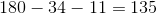Draw out the figure: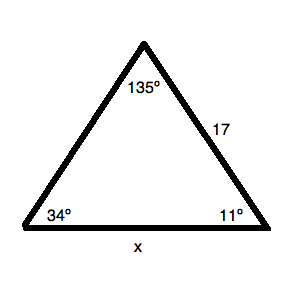Now, to solve this, you will need some trigonometry. Use the Law of Sines to calculate the value: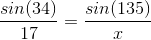Solving for, you get: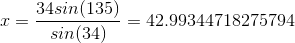Rounding, this is.

### Example Question #2 : How To Find The Length Of The Side Of An Acute / Obtuse Triangle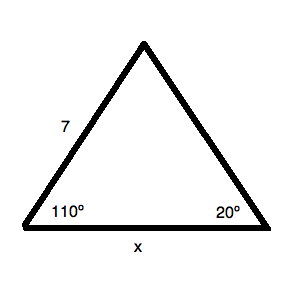What is the value ofin the triangle above? Round to the nearest hundredth.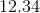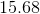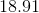Cannot be computed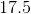Explanation:

Begin by filling in the missing angle for your triangle. Since a triangle has a total ofdegrees, you know that the missing angle is: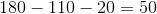Draw out the figure: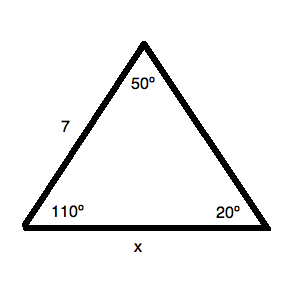Now, to solve this, you will need some trigonometry. Use the Law of Sines to calculate the value: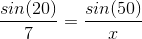Solving for, you get: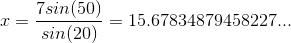Rounding, this is.

### Example Question #3 : How To Find The Length Of The Side Of An Acute / Obtuse Triangle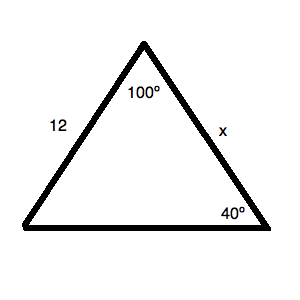What is the length of side? Round to the nearest hundredth.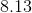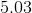Cannot be computedExplanation:

Begin by filling in the missing angle for your triangle. Since a triangle has a total ofdegrees, you know that the missing angle is: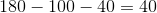Draw out the figure: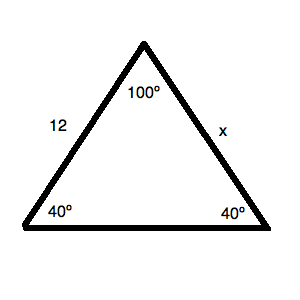This problem becomes incredibly easy! This is an isosceles triangle. Therefore, you know thatis, because it is "across" from adegree angle—which matches the otherdegree angle!